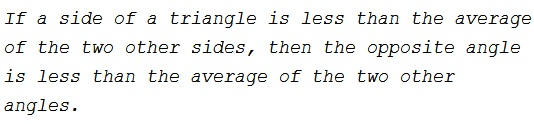# The Averages of Sides and Angles in a Triangle

### Problem### Solution 1

Since $A+B+C=180^{\circ},$ proving that $\displaystyle A\lt\frac{B+C}{2}$ amounts to proving that $\displaystyle A\lt\frac{180^{\circ}-A}{2},$ or $A\lt 60^{\circ}.$ But $A\lt 60^{\circ}$ is equivalent to $\displaystyle \cos A\gt\frac{1}{2}.$

By hypothesis, $b+c\gt 2a.$ Squaring both sides and applying the Law of Cosines, we obtain

$b^2+2bc+c^2\gt 4(b^2+c^2-2bc\cos A)$

which is equivalent to

$4bc(2\cos A-1)\gt 3(b-c)^2\ge 0,$

proving $\cos A\gt\displaystyle \frac{1}{2}.$

### Solution 2

Take an horizontal side $BC=2$ and $A$ above line $BC.$ According to the condition on the sides, $A$ lies on an ellipse with foci $B$ and $C$ and major axis greater than $4.$ The half minor axis is thus longer than the square root of $3.$ The circumcircle of $\Delta ABC$ cuts thus the line of the minor axis above $\sqrt{3},$ angle $A$ is smaller than $60^{circc}.$

### Solution 3

Let $\displaystyle a\lt \frac{b+c}{2}$.

\displaystyle \begin{align} \cos A &= \frac{b^2+c^2-a^2}{2bc} > \frac{b^2+c^2-\frac{(b+c)^2}{4}}{2bc} \\ \Rightarrow \cos A &\gt \frac{1}{2}+\frac{3}{8bc}\cdot\left[(b^2+c^2)-2bc\right] \\ \Rightarrow \cos A &\gt \frac{1}{2} = \cos 60^{\circ}.~\text{(AM-GM)} \end{align}

Thus, $A\lt 60^{\circ}$ and $\displaystyle \frac{B+C}{2}=90^{\circ}-\frac{A}{2}\gt 60^{\circ}$, implying $\displaystyle A\lt \frac{B+C}{2}$.

### Acknowledgment

The problem and the solution came from The Stanford Mathematics Problem Book by G. Polya and J. Killpatrick (52.1). Solution 2 is by Grégoire Nicollier; Solution 3 is by Amit Itagi.